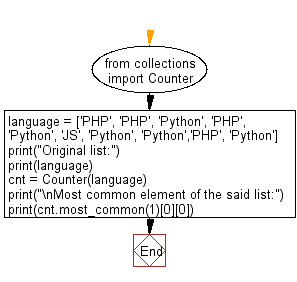﻿ Python: Find the most common element of a given list - w3resource# Python: Find the most common element of a given list

## Python Collections: Exercise-15 with Solution

Write a Python program to find the most common element of a given list.

Sample Solution:

Python Code:

``````from collections import Counter
language = ['PHP', 'PHP', 'Python', 'PHP', 'Python', 'JS', 'Python', 'Python','PHP', 'Python']
print("Original list:")
print(language)
cnt = Counter(language)
print("\nMost common element of the said list:")
print(cnt.most_common(1))
```
```

Sample Output:

```Original list:
['PHP', 'PHP', 'Python', 'PHP', 'Python', 'JS', 'Python', 'Python', 'PHP', 'Python']

Most common element of the said list:
Python
```

Flowchart:## Visualize Python code execution:

The following tool visualize what the computer is doing step-by-step as it executes the said program:

Python Code Editor:

Have another way to solve this solution? Contribute your code (and comments) through Disqus.

What is the difficulty level of this exercise?

Test your Programming skills with w3resource's quiz.

﻿

## Python: Tips of the Day

The Zip() Function:

```>>> students = ('John', 'Mary', 'Mike')
>>> ages = (15, 17, 16)
>>> scores = (90, 88, 82, 17, 14)
>>> for student, age, score in zip(students, ages, scores):
...     print(f'{student}, age: {age}, score: {score}')
...
John, age: 15, score: 90
Mary, age: 17, score: 88
Mike, age: 16, score: 82
>>> zipped = zip(students, ages, scores)
>>> a, b, c = zip(*zipped)
>>> print(b)
(15, 17, 16)
```